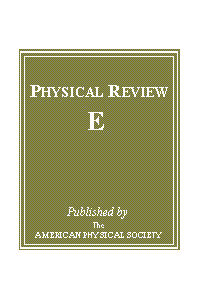## What are you looking for?# Coupling of link- and node-ordering in the coevolving voter model

## Abstract

We consider the process of reaching the final state in the coevolving voter model. There is a coevolution of state dynamics, where a node can copy a state from a random neighbor with probabilty 1-p and link dynamics, where a node can rewire its link to another node of the same state with probability p. That exhibits an absorbing transition to a frozen phase above a critical value of rewiring probability. Our analytical and numerical studies show that in the active phase mean values of magnetization of nodes n and links m tend to the same value that depends on initial conditions. In a similar way mean degrees of spins up and spins down become equal. The system obeys a special statistical conservation law since a linear combination of both types magnetizations averaged over many realizations starting from the same initial conditions is a constant of motion: Λ≡(1-p)μm(t)+pn(t)=const., where μ is the mean node degree. The final mean magnetization of nodes and links in the active phase is proportional to Λ while the final density of active links is a square function of Λ. If the rewiring probability is above a critical value and the system separates into disconnected domains, then the values of nodes and links magnetizations are not the same and final mean degrees of spins up and spins down can be different.